#### Chart with confidence intervals

In this article we will try to learn the method for creating charts with confidence interval in Excel.

## Adding confidence intervals for a graph

In Microsoft Excel, you can create a chart with confidence intervals to display the range of values that are likely to contain the true value of a set of data with a certain level of confidence. Here’s how to create such a chart:

Start by creating sample sales data for a week. Now let us create more data using stdev functions and it will show up as follows: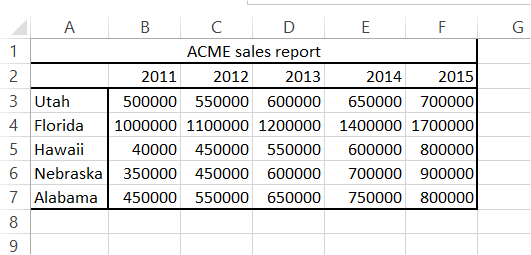Let us calculate the differences (upper band and lower band) as follows:

Upper band formula: =B2+ B3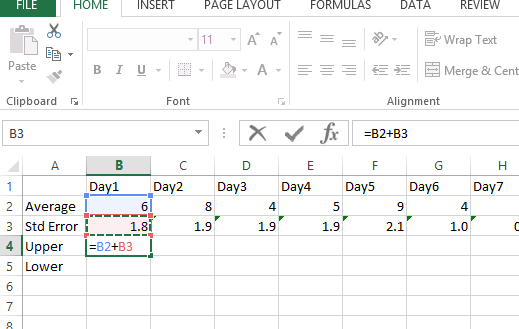Lower band formula: =B2 – B3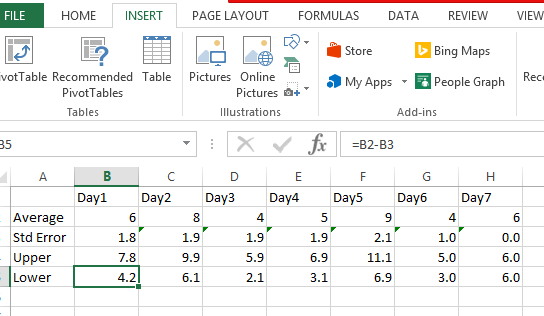## Creating a confidence interval graph

Let us try to create the 2D chart as follows: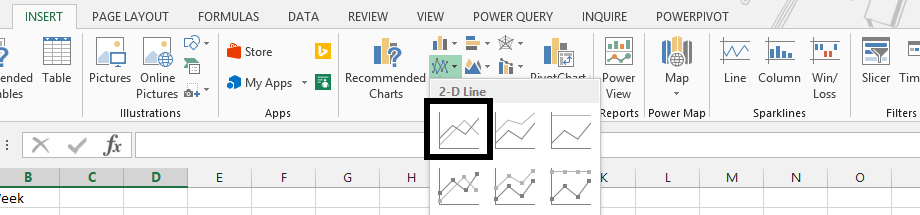Now we have to change the chart type to an area graph: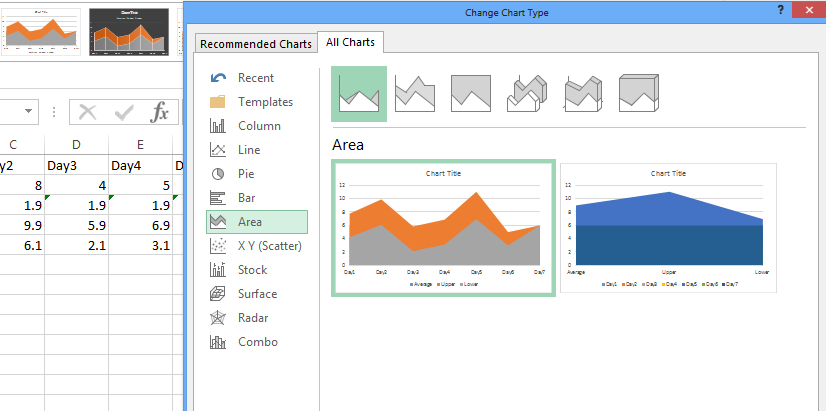It will look like this now: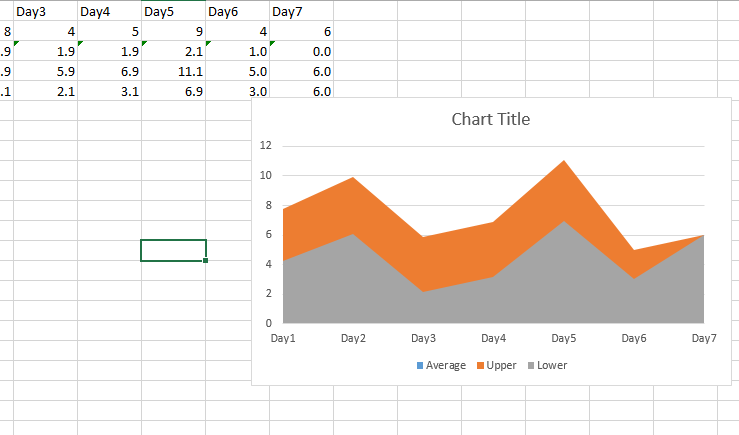To finish the chart, simply format the upper series with a light blue fill (to match the dark blue line) and the lower series with a white fill.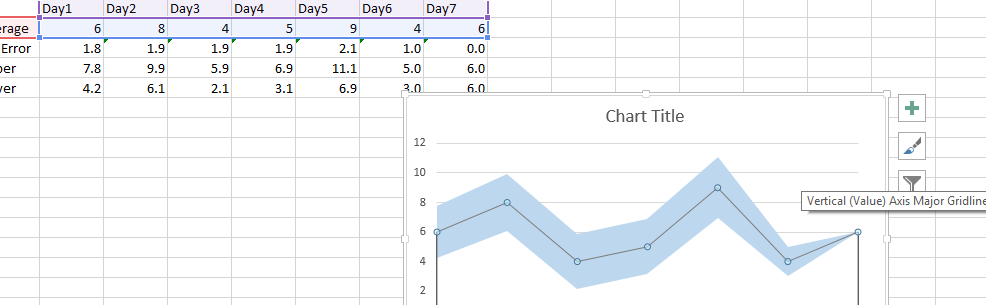Both the confidence bar chart and the confidence band chart are in the sample worksheet here. It depends upon what you prefer it to look like.

By following these steps, you can create a chart in Excel with confidence intervals, which can be a useful way to visualize and interpret data and draw conclusions from it. The chart will show the range of values that are likely to contain the true value of the data with a certain level of confidence, allowing you to make informed decisions based on the data.